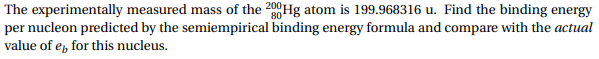# The experimentally measured mass of the 200Hg atom is 199.968316 u. Find the binding energyper nucleon predicted by the semiempirical binding energy formula and compare with the actualvalue of e for this nucleus

Question
6 viewshelp_outlineImage TranscriptioncloseThe experimentally measured mass of the 200Hg atom is 199.968316 u. Find the binding energy per nucleon predicted by the semiempirical binding energy formula and compare with the actual value of e for this nucleus fullscreen
check_circle

Step 1

Mass of proton = 1.007825 u

Mass of neutron = 1.008665 u

Mass of Hg = 199.968316 u

Mass of 80 protons = 80 × 1.007825 u = 80.626 u

Number of neutrons = 200 – 80 = 120

Mass of 120 neutrons = 120 × 1.008665 u = 121.0398 u

Total mass of 200 nucleons = 80.626 u + 121.0398 u = 201.6658 u

Mass defect of Hg, ∆m = 201.6658 – 199.968316 u = 1.697484 u

Step 2

The relation to calculate binding energy is shown as follows:

Step 3

On substituting the value of mass defect in the above rela...

### Want to see the full answer?

See Solution

#### Want to see this answer and more?

Solutions are written by subject experts who are available 24/7. Questions are typically answered within 1 hour.*

See Solution
*Response times may vary by subject and question.
Tagged in

### Physical Chemistry